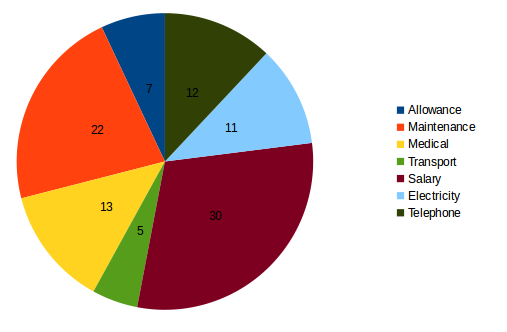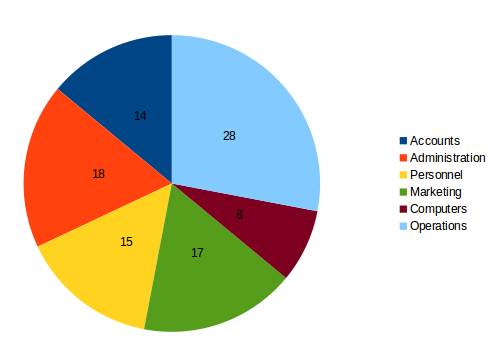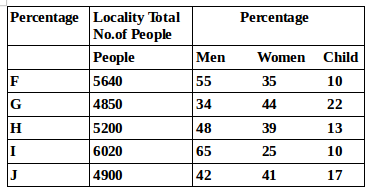## Data Interpretation For SBI PO : Set – 55

D.1-5) Study the following graph carefully to answer these questions

Details about the distribution of employees and expenditures of an organization(distributed proportionately across the departments)

Annual expenditure on different items

Total Expenditures = Rs. 12 CroresDepartment wise Distribution of Employees

Total Number of employees = 12001) What was the total expenditure on Accounts Department?

a) 16.8 millions

b) 1.680 lakhs

c) 18.6 lakhs

d) 16.8 lakhs

e) None of these

a)

Total expenditure on Accounts department = 14% of Rs. 12 crores

= Rs. (14*12)/100 crores = Rs.1.68 crorese

=Rs. 16.8 millions

2) What was per employee expenditure on Medical?

a) Rs.12000

b) Rs.13000

c) Rs. 12,500

d) Rs.13,500

e) None of these

b)

Total expenditure on Medical = 13% of Rs. 12 crores

= Rs. (13*12)/100= 1.56 Crores

Rs. 1. 56 Crores

Rs. 15600000

Expenditure per employee = 15600000/120 = Rs. 13000

3) What was the total expenditure on salary of employees in Marketing Department?

a) Rs.6.12millions

b) Rs.61.2 millions

c) Rs. 6.12 lakhs

d) Rs. 176 lakhs

e) None of these

a)

Total Expenditure on salary of employees = 30% of Rs. 12Crores

= Rs. (30*120000000)/100= Rs. 36000000

Expenditure on Marketing employees

= 17% of 36000000

Rs.(17 * 360000)

Rs. 6120000

Rs. 6.12 millions

4) What was the amout spent on Electricity?

a) Rs. 13.2 millions

b) Rs.13.2 lakhs

c) Rs.126 millions

d) Rs. 12.6 lakhs

e) None of these

a)

Amount Spent on electricity = 11% of Rs. 12 Crores

= Rs. (11*120)/100millions = Rs. 13.2 millions

5) What was the expenditure on telephone for employees in computer Department?

a) Rs.11.52 lakhs

b) Rs. 11.52 millions

c) Rs. 10.72 lakhs

d) Rs. 10.72 milions

e) None of these

b)

Expenditure on telephone in computer department = 12% o 8% of Rs. 12 Crores

= Rs. (12/100)*(8/100)*120millions

=Rs.1.152 millions

=Rs. 11.52 lakhs

D.6-10) Study the following and answer the questions:6) Total number of people staying in locality J Forms approximately what per cent of the total number of people staying in locality F?

a) 81

b) 72

c) 78

d) 93

e) 87

e)

Required % = (490/564)*100= 87%

7) What is the total  number of children staying in localities H and I together?

a) 1287

b) 1278

c) 1827

d) 1728

e) None of these

b)

Required number of children = 52 * 13 + 602 =1278

8) The number of women staying in which locality is the highest?

a) H

b) J

c) F

d) G

e) None of these

d)

Number of women living in the locality

F : 56.4 * 35 = 56 * 35 +0.4 * 35 =1960+14 =1974

G: 48.5 * 44= 2112+222 = 2134

H : 52 * 39 = 2028

I : 6020 *1/4 = 1505

J : 49 * 41 = 2009

9) What is the total number of men and children staying in locality I together?

a) 4115

b) 4551

c) 4515

d) 4155

e) None of these

c)

Total number of men and children in locality ,

I =6020 * (65+10)/100 = 6020 * 3/4 = 4515

10) What is the ratio of the number of men staying in locality F to the number of men staying in locality H?

a) 517:416

b) 403:522

c) 416:517

d) 522:403

e) None of these